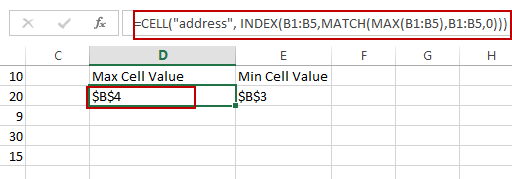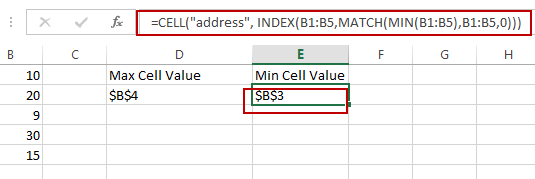# How to Get Cell Address of Max or Min Value in a Range in Excel

This post will guide you how to return the cell address of a maximum cell value from a given range of cells with a formula in Excel. How do I Find the minimum value from a given range and return its cell address with formula in Excel 2010/2013/2016.

## Return Cell Address of Max Value in a Range

Assuming that you have a list of data in range B1:B5, which contain some numbers, and you need to find the largest value from this range, and return the cell address. How to do it. You can use a formula based on the CELL function, the INDEX function, the MATCH function and the MAX function to get the cell address of the largest value in range. Like this:

`=CELL("address", INDEX(B1:B5,MATCH(MAX(B1:B5),B1:B5,0)))`

Type this formula into a blank cell and press Enter key on your keyboard. It would return the cell address of the maximum value in range B1:B5.You can change the range B1:B5 as you need to get the cell address of the maximum value in a list.

## Return Cell Address of Min Value in a Range

If you want to find the smallest value in range B1:B5, and return the cell address. You can use the following formula based on the CELL function, the INDEX function, the MATCH function and the MIN function. Like this:

`=CELL("address", INDEX(B1:B5,MATCH(MIN(B1:B5),B1:B5,0))) `

Type this formula into a blank cell and press Enter key on your keyboard. It would return the cell address of the minimum value in range B1:B5.Let’s see how those two formula work:

The INDEX/MATCH function will return the reference of the Maximum or minimum value from a range. And the Cell function returns the cells address。

### Related Functions

• Excel INDEX function
The Excel INDEX function returns a value from a table based on the index (row number and column number)The INDEX function is a build-in function in Microsoft Excel and it is categorized as a Lookup and Reference Function.The syntax of the INDEX function is as below:= INDEX (array, row_num,[column_num])…
• Excel MATCH  function
The Excel MATCH function search a value in an array and returns the position of that item.The MATCH function is a build-in function in Microsoft Excel and it is categorized as a Lookup and Reference Function.The syntax of the MATCH function is as below:= MATCH  (lookup_value, lookup_array, [match_type])….
• Excel CELL function
The Excel CELL function returns information about the formatting, location, size, or contents of a cell.The syntax of the CELL function is as below:= CELL (info_type,[reference])…
• Excel MIN function
The Excel MIN function returns the smallest numeric value from the numbers that you provided. Or returns the smallest value in the array.The MIN function is a build-in function in Microsoft Excel and it is categorized as a Statistical Function.The syntax of the MIN function is as below:= MIN(num1,[num2,…numn])….
• Excel MAX function
The Excel MAX function returns the largest numeric value from the numbers that you provided. Or returns the largest value in the array.= MAX(num1,[num2,…numn])…